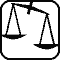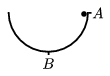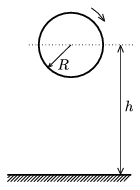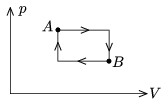Mathematical and Physical Journal
for High Schools
Issued by the MATFUND Foundation
 Already signed up? New to KöMaL?

# Exercises and problems in PhysicsOctober 2004## Experimental problem

M. 254. Measure the density of both a dry and a soaking wet skewer stick. Examine the time dependence of the density of the stick when placed into water.

(6 points)## Theoretical problems

It is allowed to send solutions for any number of problems, but final scores of students of grades 9-12 are computed from the 5 best score in each month. Final scores of students of grades 1-8 are computed from the 3 best scores in each month.

P. 3732. An empty cube of an edge length of a=10 cm and wall thickness of d=2 cm is floating in water with half of its volume immersed. What is the density of the material its wall is made of?

(3 points)

P. 3733. If we leave a metal barrel open in the garden for a few years then its bottom gets spherical. Why?

(3 points)

P. 3734. A small ball can move in a vertical plane along a semi-circle of radius R without friction. At what speed is the ball to launch from point A so that its acceleration is 3g at point B? Where along its path is its acceleration horizontal? What is the acceleration here?(5 points)

P. 3735. If at sunset we see a half-moon from the Earth, what does the Earth look like from the Moon at the same time?

(4 points)

P. 3736. h=0.95 m above a horizontal surface we spin a rim of a radius of R=0.2 m at an angular velocity of $\displaystyle \omega$0=5 s-1 and release it without any initial velocity. After colliding with the horizontal surface the rim leaves the surface at a velocity of v=2 m/s and angular velocity of $\displaystyle \omega$1=3 s-1. a) What percentage of its kinetic energy is lost in the collision? b) At what angle does the rim leave the horizontal surface?(5 points)

P. 3737. We start two pendulum-clocks at the same time. Of the two only one is accurate, and at its 777th swing the two clocks swing together again. By what percentage do we have to change the length of the inaccurate pendulum to make it accurate?

(4 points)

P. 3738. A square shaped copper plate is lying on a roof. It does not slip because the friction is quite high. During the day the temperature is higher and at night it is lower, therefore the plate expands and contracts. Estimate the distance it moves from its place in one year. Data: The length of the side of the square is 1 meter, the slope angle of the roof is 30o, the coefficient of friction is 1 and the plate presses the roof uniformly. The average difference in the day and night temperature is 10 oC and the thermal expansion of the roof is negligible.

(5 points)

P. 3739. We make helium gas of 2 grams to go through the thermal cycle shown in the figure. The lowest temperature of the gas in the cycle is 16 oC, the highest is 88 oC, and in the meantime the temperature is equal at points A and B. a) What is the gas temperature at points A and B? b) How much work is done by the gas in this thermal cycle? c) How much heat is absorbed and transmitted in one cycle by the gas?(5 points)

P. 3740. What is the ratio of the electrostatic potentials on the surface and at the center point of a uniformly charged sphere? (The potential is considered 0 at infinity.)

(4 points)

P. 3741. What is the ratio of the electrostatic potentials at one corner and in the center point of a uniformly charged cube? (The potential is considered 0 at infinity.)

(5 points)

P. 3742. From a point sound source the power of the energy outflow is P. The sound velocity is v. What are the density of energy and the energy flux (energy flowing across unit surface in unit time) in r distance of the source? How does the amplitude depend on the position?

(4 points)